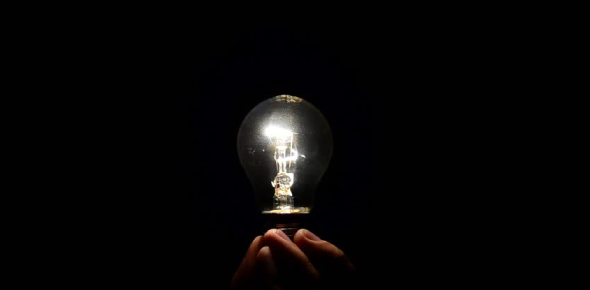# Electricity Quiz: How Much You Know? Trivia Questions

10 Questions | Total Attempts: 82SettingsWould you like to learn about electricity? This quiz may be of assistance. This quiz encourages you to ascertain what is a simple device that opens and closes an electrical unit, the unit used for measuring electrical current, what a path without brakes is called, and what materials do not allow electrical charges to pass through easily. This phenomenal quiz will help you to learn more about electricity.

• 1.
A simple device that opens and closes an electrical unit is called a(an):
• A.

Volt

• B.

Discharge

• C.

Switch

• D.

Ampere

• 2.
The unit used for measuring electrical current is called a(an):
• A.

Electrode

• B.

Volt

• C.

Discharge

• D.

Ampere

• 3.
This is the unit of measurement for measuring electrical pressure or EMF?
• A.

Switch

• B.

Volt

• C.

Ampere

• D.

Electrode

• 4.
Material that electricity can travel through easily is called a
• A.

Conductor

• B.

Insulator

• C.

Semiconductor

• D.

None of these

• 5.
A circuit is a path taken by a current. A path with no breaks is called a
• A.

Closed circuit

• B.

Open circuit

• C.

Open switch

• D.

None of these

• 6.
If there is a break in the path the current follows, the circuit is incomplete. A break in the path is called an
• A.

Open circuit

• B.

Close circuit

• C.

Both A and B

• D.

None of the above

• 7.
Materials that do not allow electrical charges to pass through it easily are called
• A.

Conductor

• B.

Insulator

• C.

Semiconductors

• D.

All of the above

• 8.
Lightning is caused by the build-up of electrical charges in a cloud, also known as static electricity.
• A.

True

• B.

False

• 9.
Parallel circuits have only one path.
• A.

True

• B.

False

• 10.
The current always travels from the negative electrode to the positive electrode.
• A.

True

• B.

False

Related TopicsBack to top